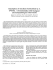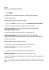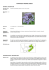# 12.2 Square Roots CORD Math Mrs. Spitz Spring 2007

## Transcription

12.2 Square Roots CORD Math Mrs. Spitz Spring 2007
```12.2 Square Roots
CORD Math
Mrs. Spitz
Spring 2007
Objectives
• Simplify rational square
roots, and
• find approximate values for
square roots
Assignment
• pp. 479-480 #5-51 all
• 82 is read “eight squared
and means eight is used as
a factor two times.
• -8 is used as a factor two
times.
• The opposite of squaring a
number is finding its square
root. To find the square
root of 64, you must find
two EQUAL factors whose
product is 64.
• Since 8 times 8 is 64, one
square root is 8. Since -8
times -8 is also 64, another
square root of 64 is -8.
Definition of a Square Root
• If x2 = y, then x is a square
root of y.
INDEX
3
64
What do these mean?
64  8
 64  8
indicate the principal square
root of 64.
indicates the negative square
root of 64.
 64  8
indicates both square roots of
64. Note this is read, “plus or
minus the square root of 64.”
Ex. 1: Find each square root.
81
Since 92 = 81, you know that
the square root is 9.
Ex. 2: Find each square root.
 0.09 
Since (0.3)2 = 0.09, you
know that the square root is
±0.3.
What if it doesn’t go evenly?
• Sometimes you may need
to use prime factorization
to find a square root of a
number.
Ex. 3: Find each square root.
 576 
576  2  2  2  2  2  2  3  3
 2 3
6
2
 (2  3)
3
 24
2
2
Since 242 = 576, you know
that the negative square root
is -24.
Ex. 4: Find each square root.
8
256
2
 4 2
2025 3  5
4
2 2
( 2 )
3 5
16 2
( )
45
16

45
Calculators
• If you happen to own one, and
you should. I would highly
recommend the TI-83 or 84 if
you are going to continue in
mathematics. If not, the TI30IIXS will suffice. It’s about \$15 at
Wal-mart. In any case, you will
need one if you are doing
textbook on page 645 which
has a table of values.
Ex. 5. Find the square root of:
2209
Square root – 1st row 6 buttons
down.
Enter the number 2209 and click
enter or =
The value should be 47.
Ex. 6. Find the square root of:
 1236
Square root – 1st row 6 buttons
down.
Enter the number 1236 and click
enter or =
The value should be 35.1567917
```

### a TRIP- Order Form Oct 6 09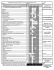### Roots 1 - Macmillan/McGraw-Hill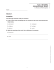### Derrick Henry Lehmer, “A Cross-Division Process and Its Application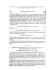### Section 6_1 Worksheet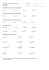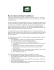### A Successful Formula, Down to the T-Bone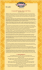### 3.3 The Factor Theorem Factor Theorem: If a polynomial () is divided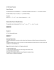### tHEy CAN`t DENy Us.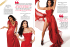### CaRoot 325 - CaPlast Kunststoffverarbeitungs GmbH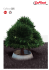### Sample Question Paper - Class - X MATHEMATICS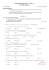### How To Act Like A Leader Presented by: Marcia Donlon RN,BSN,MS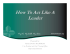### Goutweed (Aegopodium podagraria)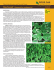### the little brown church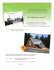### Holiday Home Work Class XI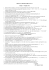### Squares, Square Roots and other radicals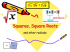### 4/20/15 - Mrs. Regal Coller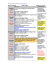### WORKSHEET 9-5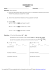### Worksheet #15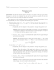### Signals and Systems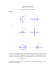### as a PDF